# Chapter 7: Timestamped data¶

Accompanying code for the book The Art of Feature Engineering.

This notebook plus notebooks for the other chapters are available online at https://github.com/DrDub/artfeateng

Permission is hereby granted, free of charge, to any person obtaining a copy of this software and associated documentation files (the "Software"), to deal in the Software without restriction, including without limitation the rights to use, copy, modify, merge, publish, distribute, sublicense, and/or sell copies of the Software, and to permit persons to whom the Software is furnished to do so, subject to the following conditions:

The above copyright notice and this permission notice shall be included in all copies or substantial portions of the Software.

THE SOFTWARE IS PROVIDED "AS IS", WITHOUT WARRANTY OF ANY KIND, EXPRESS OR IMPLIED, INCLUDING BUT NOT LIMITED TO THE WARRANTIES OF MERCHANTABILITY, FITNESS FOR A PARTICULAR PURPOSE AND NONINFRINGEMENT. IN NO EVENT SHALL THE AUTHORS OR COPYRIGHT HOLDERS BE LIABLE FOR ANY CLAIM, DAMAGES OR OTHER LIABILITY, WHETHER IN AN ACTION OF CONTRACT, TORT OR OTHERWISE, ARISING FROM, OUT OF OR IN CONNECTION WITH THE SOFTWARE OR THE USE OR OTHER DEALINGS IN THE SOFTWARE.

## Limitations¶

• Simple python intented for people coming from other languages that plan to use the ideas described in the book outside of Python.
• Many of these techniques are available as library calls. They are spelled out as for teaching purposes.
• Resource limitations:
• At most one day of running time per notebook.
• No GPU required.
• Minimal dependencies.
• At most 8Gb of RAM.
• Due to resource limitations, these notebooks do not undergo as much hyperparameter tuning as necessary. This is a shortcoming of these case studies, keep it in mind if you want to follow a similar path with your experiments.
• To help readers try variants of some cells in isolation, the cells are easily executable without having to re-run the whole notebook. As such, most cells read everything they need from disk and write all their results back into disk, which is unnecessary with normal notebooks. The code for each cell might look long and somewhat unusual. In a sense, each cell tries to be a separate Python program.
• I dislike Pandas so these notebooks are Pandas-free, which might seem unusual to some.

# Chapter 7: Case Study on Timestamped Data¶

In this chapter I will describe the creation of the extended version of WikiCities dataset with historical data. I will also present a country population prediction task to exemplify traditional time series algorithms.

## WikiCities: Historical¶

Used the following historical versions of DBpedia:

VersionYearCode
3.62010dbpedia10
3.72011dbpedia11
3.82012dbpedia12
3.92013dbpedia13
20142014dbpedia14
2015-042015dbpedia15

Using scripts outside of this notebook, I have extracted the relevant triples from the dumps for all years into files CODE_cities1000_base.ttl (e.g., dbpedia12_cities1000_base.ttl). Because due to changes in the script (going from or "Phoenix%2C_Arizona" to "Phoenix,Arizona") and in the source Wikipedia (going from "Utrecht" to "Utrecht%28city%29"), the entity designators change from year to year, I have tried to standardize them using the provided GeoNames link for each year. In the historical versions the GeoNames data was limited and as many as half the cities are missing. This might also be due to lower coverage in the source Wikipedia in the earlier years. All in all, this represents the type of challenges of dealing with historical data in production systems.

From these files, I extracted the conservative feature set into CODE_dev_conservative.tsv, also outside the notebooks. This produced files CODE_dev_conservative.tsv. Again, due to changes in the ontology many columns (sometimes up to 50% of the columns) are empty. Interestingly, this number changes from year to year (not necessarily starting low and increasing). Therefore, it enables the specific type of temporal imputation enabled by timestamped data.

Let us start doing some EDA and see how this data set behaves.

## EDA: Exploratory Data Analysis¶

The first thing to take a look is how the total number of relations behaves year after year (Cell #1).

In :
# CELL 1
import math
import numpy as np
import matplotlib.pyplot as plt
%matplotlib inline

dbpedia_rels = [
19969604, # 2010
26770236, # 2011
33742028, # 2012
41804713, # 2013
61481509, # 2014
37791134, # 2015
38285149 # 2016
]

city_col = 0
rel_col = 1
to_plot = list()
means = list()
city_to_data = None
deleted_cities = set()
for version in range(7):
datafile = "dbpedia1" + str(version) + "_dev_conservative.tsv"
if version == 6:
datafile = "ch6_cell32_dev_feat_conservative.tsv"
city_relcounts = dict()
with open(datafile) as f:
first = True
for line in f:
fields = line.split("\t")
if first:
first = False
if fields[city_col] != 'name':
raise Exception("Expected 'name', got " + fields[city_col])
if fields[rel_col] != 'rel#count':
raise Exception("Expected 'rel#count', got " + fields[rel_col])
else:
city_relcounts[fields[city_col]] = float(fields[rel_col])
if city_to_data is None:
city_to_data = dict()
for city, rel in city_relcounts.items():
city_to_data[city] = [ rel ]
else:
to_delete = list()
for city, l in city_to_data.items():
if city in city_relcounts:
l.append(city_relcounts[city])
else:
to_delete.append(city)
for city in to_delete:
del city_to_data[city]
print("Plotting on {:,}".format(len(city_to_data)))
cities = sorted(list(city_to_data.keys()))
for version in range(7):
relcounts = np.array([ city_to_data[city][version] for city in cities])
to_plot.append(relcounts)
means.append(np.mean(relcounts))
fig, ax = plt.subplots()
fig.set_size_inches(15, 15)
ax.set_title('Total relation log counts by year')
ax.boxplot(to_plot, labels=list(range(2010,2017)), meanline=True)
means.insert(0,0)
ax.plot(range(8), means, 'b-', color='gray')
ax2 = ax.twinx()
ax2.plot(list(map(math.log,dbpedia_rels)), linestyle=(0,(5,10)), color='black', linewidth=3.0)
plt.savefig("ch7_cell1_boxplot.pdf", bbox_inches='tight', dpi=300)
plt

Plotting on 44,959

Out:
<module 'matplotlib.pyplot' from 'feateng/lib/python3.7/site-packages/matplotlib/pyplot.py'>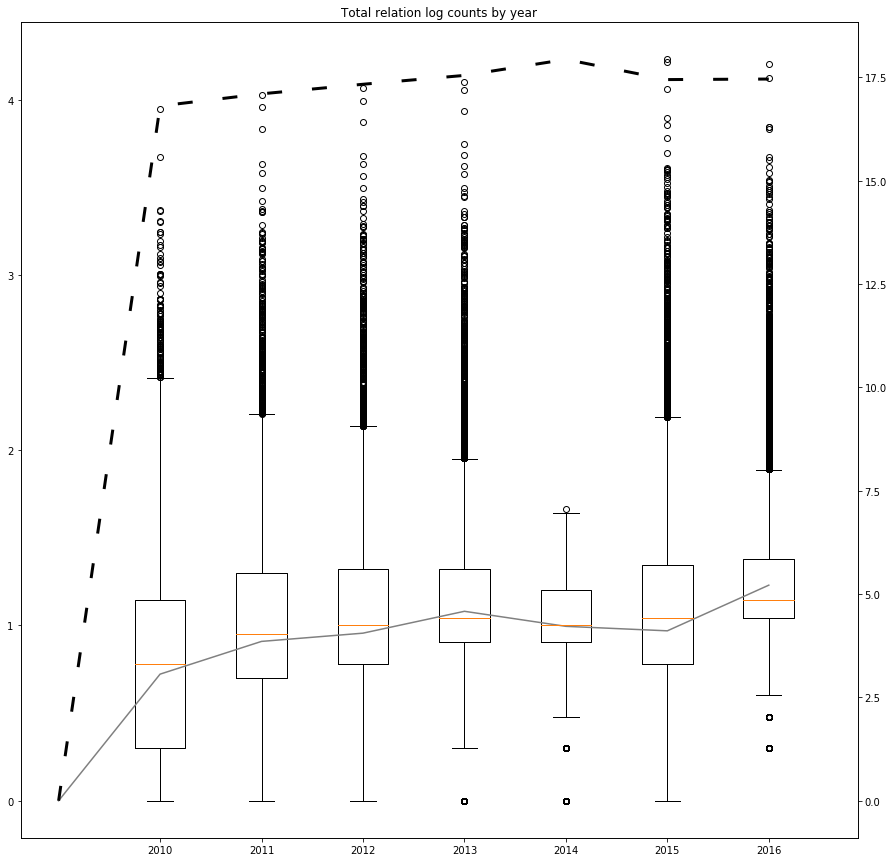Interestingly, the average number of relations has not increased as much as the average increase of DBpedia.

Let us see the behaviour of this number as related to the last year (Cell #2).

In :
# CELL 2
import numpy as np
import matplotlib.pyplot as plt
%matplotlib inline

city_col = 0
rel_col = 1
to_plot = list()
means = list()
city_to_data = None
deleted_cities = set()
for version in range(7):
datafile = "dbpedia1" + str(version) + "_dev_conservative.tsv"
if version == 6:
datafile = "ch6_cell32_dev_feat_conservative.tsv"
city_relcounts = dict()
with open(datafile) as f:
first = True
for line in f:
fields = line.split("\t")
if first:
first = False
if fields[city_col] != 'name':
raise Exception("Expected 'name', got " + fields[city_col])
if fields[rel_col] != 'rel#count':
raise Exception("Expected 'rel#count', got " + fields[rel_col])
else:
city_relcounts[fields[city_col]] = float(fields[rel_col])
if city_to_data is None:
city_to_data = dict()
for city, rel in city_relcounts.items():
city_to_data[city] = [ rel ]
else:
to_delete = list()
for city, l in city_to_data.items():
if city in city_relcounts:
l.append(city_relcounts[city])
else:
to_delete.append(city)
for city in to_delete:
del city_to_data[city]
print("Plotting on {:,}".format(len(city_to_data)))
cities = sorted(list(city_to_data.keys()))
for version in range(7):
relcounts = np.array([ city_to_data[city][version] - city_to_data[city][-1] for city in cities ])
to_plot.append(relcounts)
means.append(np.mean(relcounts))
fig, ax = plt.subplots()
fig.set_size_inches(15, 15)
ax.set_title('Difference relation log counts to 2016 by year')
ax.boxplot(to_plot, labels=list(range(2010,2017)), meanline=True)
means.insert(0,0)
ax.plot(range(8), means, 'b-', color='gray')
plt.savefig("ch7_cell2_boxplot.pdf", bbox_inches='tight', dpi=300)
plt

Plotting on 44,959

Out:
<module 'matplotlib.pyplot' from 'feateng/lib/python3.7/site-packages/matplotlib/pyplot.py'>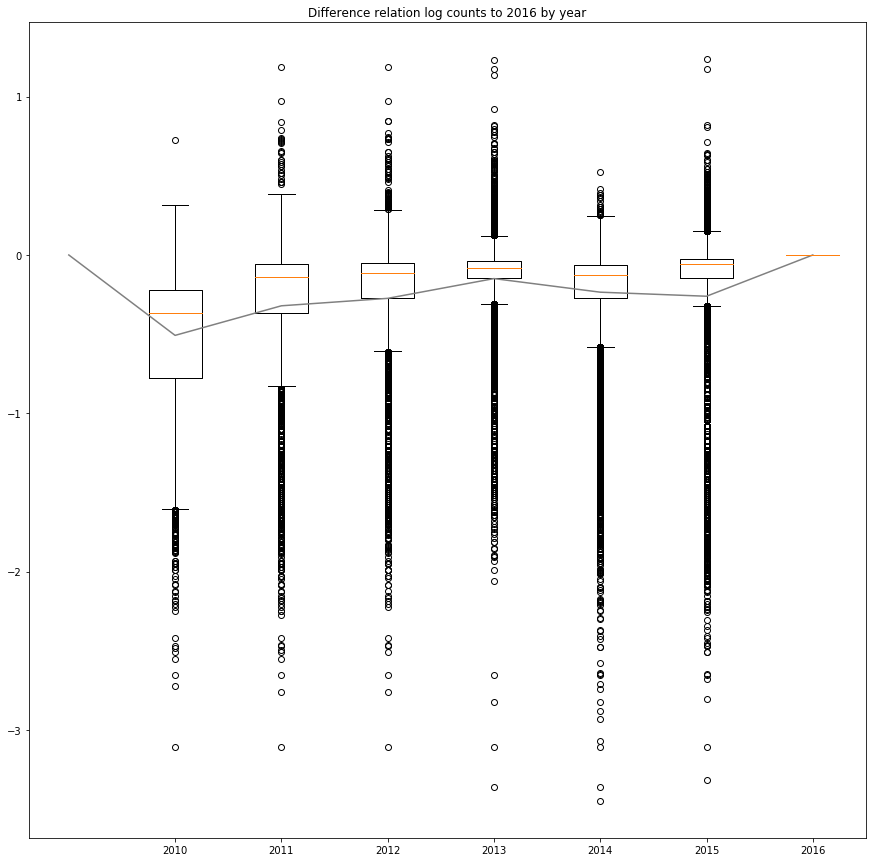Let us now take a look at the number of different features to the current year (Cell #3).

In :
# CELL 3
import numpy as np
import matplotlib.pyplot as plt
%matplotlib inline

city_col = 0
to_plot = list()
means = list()
city_to_data = None
deleted_cities = set()
for version in range(7):
datafile = "dbpedia1" + str(version) + "_dev_conservative.tsv"
if version == 6:
datafile = "ch6_cell32_dev_feat_conservative.tsv"
this_city_data = dict()
with open(datafile) as f:
for line in f:
fields = line.split("\t")
this_city_data[fields[city_col]] = list(map(float,fields[1:]))
if city_to_data is None:
city_to_data = dict()
for city, data in this_city_data.items():
city_to_data[city] = [ data ]
else:
to_delete = list()
for city, l in city_to_data.items():
if city in this_city_data:
l.append(this_city_data[city])
else:
to_delete.append(city)
for city in to_delete:
del city_to_data[city]
print("Plotting on {:,}".format(len(city_to_data)))
cities = sorted(list(city_to_data.keys()))
for version in range(7):
equal_counts = list()
for city in cities:
equal_count = 0
for idx in range(len(city_to_data[city][-1])):
if city_to_data[city][version][idx] == city_to_data[city][-1][idx]:
equal_count += 1
equal_counts.append(equal_count)
equal_counts = np.array(equal_counts)
to_plot.append(equal_counts)
means.append(np.mean(equal_counts))
fig, ax = plt.subplots()
fig.set_size_inches(15, 15)
ax.set_title('Equal features to 2016 by year')
ax.boxplot(to_plot, labels=list(range(2010,2017)), meanline=True)
means.insert(0,0)
ax.plot(range(8), means, 'b-', color='gray')
plt.savefig("ch7_cell3_boxplot.pdf", bbox_inches='tight', dpi=300)
plt

Plotting on 44,959

Out:
<module 'matplotlib.pyplot' from 'feateng/lib/python3.7/site-packages/matplotlib/pyplot.py'>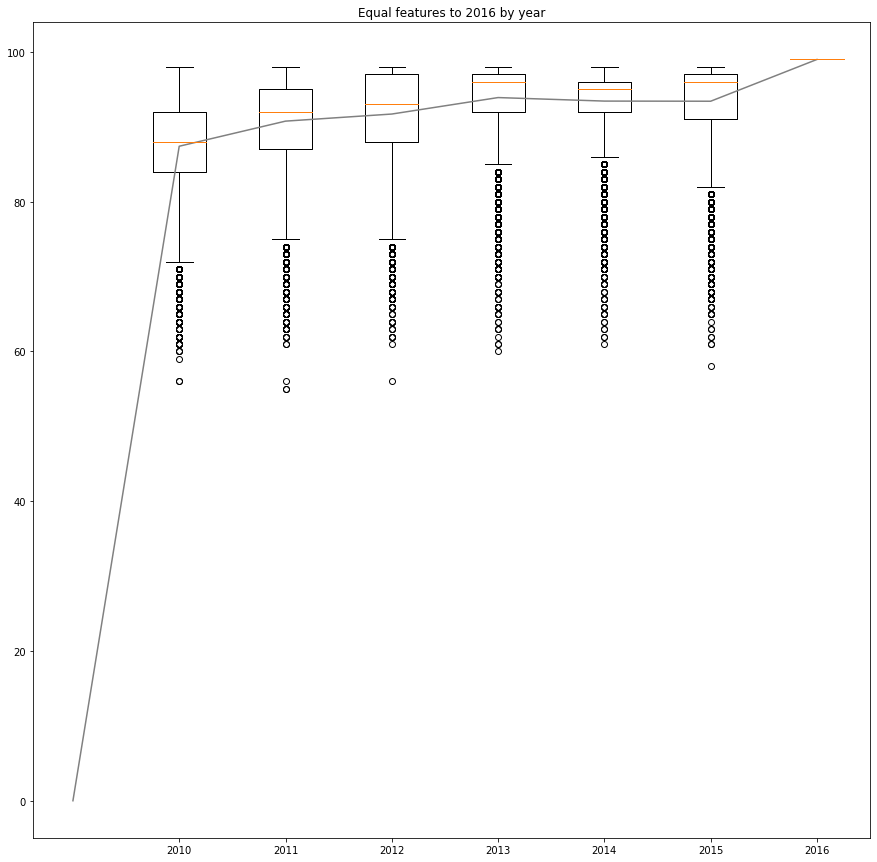The trend is similar to the one observed in the rel count alone. This data seems reasonable for trying lagged features but temporal smoothing using a sliding window might provide better results given the bouncy behaviour of the data.

To conclude the EDA, let us take a look at the behaviour of the difference between the target variable, population (Cell #4).

In :
# CELL 4
import numpy as np
import matplotlib.pyplot as plt
%matplotlib inline

city_col = 0
pop_col = 99
to_plot = list()
means = list()
city_to_data = None
deleted_cities = set()
for version in range(7):
datafile = "dbpedia1" + str(version) + "_dev_conservative.tsv"
if version == 6:
datafile = "ch6_cell32_dev_feat_conservative.tsv"
city_pop  = dict()
with open(datafile) as f:
first = True
for line in f:
fields = line.strip().split("\t")
if first:
first = False
if fields[city_col] != 'name':
raise Exception("Expected 'name', got " + fields[city_col])
if fields[pop_col] != 'population':
raise Exception("Expected 'population', got " + fields[pop_col])
else:
city_pop[fields[city_col]] = float(fields[pop_col])
if city_to_data is None:
city_to_data = dict()
for city, rel in city_pop.items():
city_to_data[city] = [ rel ]
else:
to_delete = list()
for city, l in city_to_data.items():
if city in city_pop and city_pop[city] > 0:
l.append(city_pop[city])
else:
to_delete.append(city)
for city in to_delete:
del city_to_data[city]
print("Plotting on {:,}".format(len(city_to_data)))
cities = sorted(list(city_to_data.keys()))
for version in range(7):
pops = np.array([ city_to_data[city][-1] - city_to_data[city][version] for city in cities])
nonzero = np.count_nonzero(pops)
print("Year {}, different: {:,} ({:%})".format(2010+version, nonzero, nonzero / len(pops)))
to_plot.append(pops)
means.append(np.mean(pops))
fig, ax = plt.subplots()
fig.set_size_inches(15, 15)
ax.set_title('Difference in log population to 2016 by year')
ax.boxplot(to_plot, labels=list(range(2010,2017)), meanline=True)
means.insert(0,0)
ax.plot(range(8), means, 'b-', color='gray')
plt.savefig("ch7_cell4_boxplot.pdf", bbox_inches='tight', dpi=300)
plt

Plotting on 30,380
Year 2010, different: 21,054 (69.302172%)
Year 2011, different: 16,327 (53.742594%)
Year 2012, different: 12,211 (40.194207%)
Year 2013, different: 8,543 (28.120474%)
Year 2014, different: 6,207 (20.431205%)
Year 2015, different: 4,541 (14.947334%)
Year 2016, different: 0 (0.000000%)

Out:
<module 'matplotlib.pyplot' from 'feateng/lib/python3.7/site-packages/matplotlib/pyplot.py'>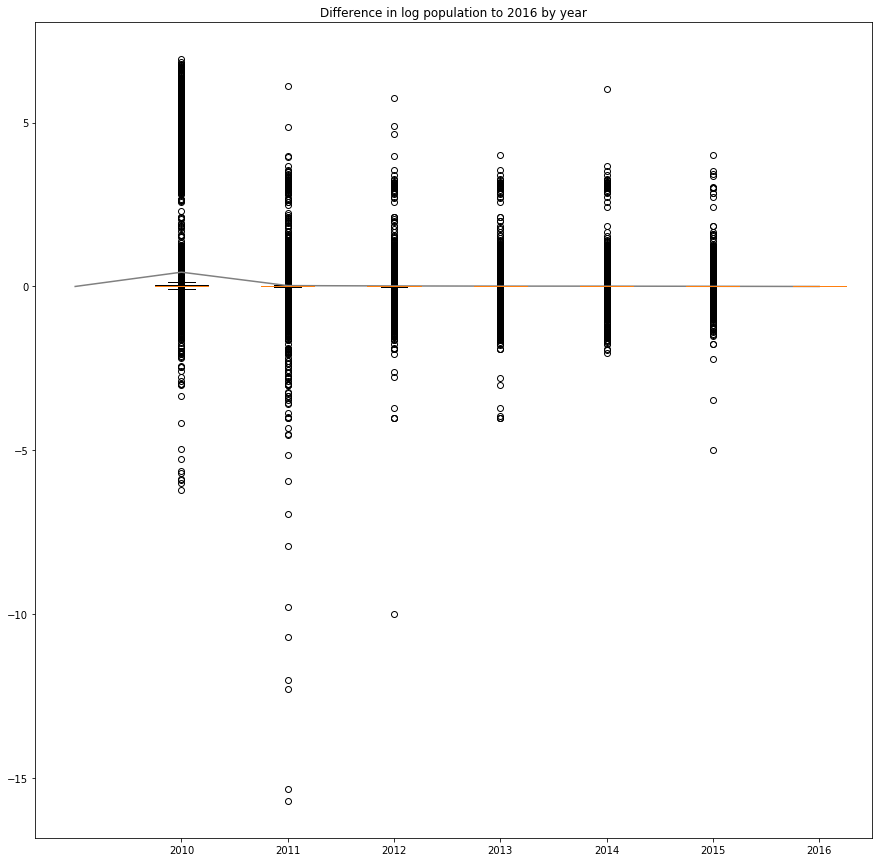Interestingly, the population numbers have changed since 2010 until 2016, even though census happen in different countries once every ten years. Also, restricting to the cities with known population for all the years does not show any bump in 2015 as in the other years. Initially, I would have expected the target population will be so accurate that giving it to the ML will constitute a target leak. While the actual number might not be the exact number, the box plot shows the number is on average the same, with some marked outliers.

Let's try to study the missing values now.

### Missing values¶

There are two sources for needs of missing values:

1. Empty relation for that year (the whole column will be empty)
2. Missing city for that year (the whole row will be empty)

I will detect these two cases, making an indicator feature for each cell based on these two sources and saving them for further imputation (Cell #5).

In :
# CELL 5
import random
import math
import numpy as np
from collections import OrderedDict

rand = random.Random(42)

# get data sizes
VERSIONS=6 # + 1 for current

with open("ch6_cell32_dev_feat_conservative.tsv") as f:
FEATS = len(next(f).split('\t')) - 1 # name
CITIES = 0
for _ in f:
CITIES += 1

CITY_COL = 0
city_names = OrderedDict() # name to idx

table = np.zeros( (CITIES, FEATS * 2 * VERSIONS + FEATS) )

with open("ch6_cell32_dev_feat_conservative.tsv") as f:
city_idx = 0
for line in f:
fields = line.strip().split("\t")
floats = list(map(float,fields[1:]))
for idx, val in enumerate(floats):
if math.isnan(val):
floats[idx] = 0.0
table[city_idx,-FEATS:] = floats
city_names[fields[CITY_COL]] = city_idx
city_idx += 1

year_defined = { city: set() for city in city_names }
for version in range(VERSIONS):
with open("dbpedia1" + str(version) + "_dev_conservative.tsv") as f:
for line in f:
fields = line.strip().split("\t")
city = fields[CITY_COL]
city_idx = city_names.get(city, None)
if city_idx is None:
continue
floats = list(map(float,fields[1:]))
for idx, val in enumerate(floats):
table[city_idx,FEATS * 2 * version + 2 * idx] = 1.0 # defined
if math.isnan(val):
table[city_idx,FEATS * 2 * version + 2 * idx + 1] = 0.0
else:
table[city_idx,FEATS * 2 * version + 2 * idx + 1] = val

# column-based missing values: determine empty/constant columns
empty_cols_per_year = list()
for version in range(VERSIONS):
empty_cols = 0
for col in range(FEATS):
value = None
constant = True
for city_idx in range(CITIES):
this_val = table[city_idx, 2 * version * FEATS + 2 * col + 1]
if value is None:
value = this_val
elif value != this_val:
constant = False
break
if constant:
empty_cols += 1
table[:, 2 * version * FEATS + 2 * col] = 0.0 # missing
empty_cols_per_year.append(empty_cols)
print("Total empty cols {:,}".format(sum(empty_cols_per_year)))
for version in range(6):
print("Empty cols for {}: {}".format(2010+version, empty_cols_per_year[version]))
print("Working on {:,} cities".format(CITIES))

# row-based imputation: determine empty years
empty_rows_per_year = list()
for version in range(6):
empty_rows = 0
for city, city_idx in city_names.items():
if version not in year_defined[city] or \
all(map(lambda x:table[city_idx, 2*version * FEATS + 2 * x + 1] == 0.0, range(FEATS))):
empty_rows += 1
for col in range(FEATS):
table[city_idx, 2 * version * FEATS + 2 * col] = 0.0
empty_rows_per_year.append(empty_rows)
print("Total empty rows {:,}".format(sum(empty_rows_per_year)))
for version in range(6):
print("Empty rows for {}: {}".format(2010+version, empty_rows_per_year[version]))

# write feature table
missing_cells = 0
total_cells = 0
with open("ch7_cell5_dev_missing.tsv", "w") as w:
w.write('name')
for version in range(VERSIONS):
w.write("\t{}_{}?is_defined".format(2010+version, featname))
w.write("\t{}_{}".format(2010+version, featname))
for city, city_idx in city_names.items():
w.write(city + "\t" + "\t".join(map(str, table[city_idx,:])) + "\n")
total_cells += FEATS * VERSIONS
for version in range(VERSIONS):
for col in range(FEATS):
if table[city_idx, 2 * version * FEATS + 2 * col] == 0.0:
missing_cells += 1
print("Total cells: {:,} missing: {:,} ({:%})".format(total_cells, missing_cells, missing_cells / total_cells))

Total empty cols 186
Empty cols for 2010: 37
Empty cols for 2011: 29
Empty cols for 2012: 29
Empty cols for 2013: 29
Empty cols for 2014: 62
Empty cols for 2015: 0
Working on 44,959 cities
Total empty rows 37,144
Empty rows for 2010: 9990
Empty rows for 2011: 8147
Empty rows for 2012: 6808
Empty rows for 2013: 2160
Empty rows for 2014: 1538
Empty rows for 2015: 8501
Total cells: 26,705,646 missing: 11,078,309 (41.483022%)


There is a significant amount of missing values. Let us see if we can visualize now individual entries. For visualization, I'll use a heatmap, discretizing features into up to six bins using k-means clustering. The top three clusters will be shown in green, the bottom in blue. Missing values will be shown in red.

The graph aligns features for each year to follow them, uncovering the story of the data (Cell #6).

In :
# CELL 6
import random
import math
import numpy as np
from sklearn.cluster import KMeans

rand = random.Random(42)

data = list()
with open("ch7_cell5_dev_missing.tsv") as f:
for line in f:
fields = line.strip().split('\t')
name = fields
feats = list(map(float, fields[1:]))
data.append( (feats, feats[-1], name) )

table = np.zeros( (len(data), len(header)) )
for idx, row in enumerate(data):
table[idx] = row

FEATS = len(base_features)
VERSIONS = 6
CITIES = len(data)

# compute boundaries using kMeans
boundaries = list()
for feat in range(FEATS):
col = table[:,2*VERSIONS*FEATS + feat]
vals = set(col)
num_clusters = min(len(vals), 6)
if num_clusters < 6:
boundaries.append(sorted(vals))
else:
kmeans = KMeans(n_clusters=6, random_state=1).fit(col.reshape(-1,1))
boundaries.append(sorted(set(list(kmeans.cluster_centers_.reshape(-1)))))

# pick some cities at random to graph
to_show = rand.sample(list(range(len(data))), 20)

def colorize(feat, feat_val):
num_classes = len(boundaries[feat])
half_classes = num_classes // 2
if half_classes == 0:
half_classes = 1
dists = sorted(map(lambda x: (abs(x-feat_val), x), enumerate(boundaries[feat])))
clazz = dists
if clazz >= half_classes:
component = 1
clazz -= half_classes
else:
component = 2
clazz = half_classes - clazz - 1

intensity = None
if clazz == 0:
if half_classes == 3:
intensity = 0.3
elif half_classes == 2:
intensity = 0.6
elif half_classes == 1:
intensity = 1.0
elif clazz == 1:
if half_classes == 3:
intensity = 0.6
elif half_classes == 2:
intensity = 1.0
elif half_classes == 1:
intensity = 1.0
elif clazz == 2:
intensity = 1.0
box_color = [ 0.0, 0.0, 0.0 ]
box_color[component] = intensity
#print(dists, clazz, num_classes, half_classes, box_color)
return box_color

def gray(box_color):
if box_color == 1.0:
col = 0.0
elif box_color == 0.0:
col = box_color / 2
else:
col = 0.5 + box_color / 2
return [ col, col, col ]

HEIGHT=2
WIDTH=2

def shrink_name(feat_name):
if '@' in feat_name:
parts = feat_name.split('@')
return parts.split("/")[-1] + "@" + parts.split("/")[-1][:-1]
else:
if '/' in feat_name:
return feat_name.split("/")[-1]
return feat_name

img = np.zeros( [ (VERSIONS + 1) * HEIGHT, FEATS * WIDTH, 3 ])
img[:,:,:] = 1.0

from matplotlib import pyplot as plt
%matplotlib inline

plt.rcParams['figure.figsize'] = [20, 40]

plt.axis('on')
yticks_locs = list(map(lambda x:x*HEIGHT, range(VERSIONS+1)))
yticks_labels = list(map(lambda x:2016-x, range(VERSIONS+1)))
xticks_locs = list(map(lambda x:x*WIDTH, range(FEATS)))
xticks_labels = list(map(lambda x:shrink_name(x), base_features))
plt.yticks(yticks_locs, yticks_labels)
plt.xticks(xticks_locs, xticks_labels, rotation=90)

idx = list(filter(lambda x:x[-1].endswith("Mérida,_Yucatán>"), enumerate(data)))
xbox = 0
for feat in range(FEATS):
ybox = feat * WIDTH
img[ xbox:(xbox + HEIGHT), ybox: (ybox + WIDTH) ] = gray(colorize(feat,
table[idx, 2 * VERSIONS * FEATS + feat]))
for version in range(VERSIONS):
xbox = (VERSIONS - version) * HEIGHT
for feat in range(FEATS):
ybox = feat * WIDTH
box_color = gray(colorize(feat, table[idx, 2 * version * FEATS + 2 * feat + 1]))
# mark imputation
if table[idx,  2 * FEATS * version + 2 * feat] == 0.0:
img[ xbox:(xbox + HEIGHT), ybox: (ybox + WIDTH) ] = [ 1.0, 1.0, 1.0 ]
img[ xbox + 1, ybox ] = [ 0.0, 0.0, 0.0 ]
img[ xbox, ybox + 1 ] = [ 0.0, 0.0, 0.0 ]
else:
img[ xbox:(xbox + HEIGHT), ybox: (ybox + WIDTH) ] = box_color
print("{}: {} ({:,} people)".format(data[idx][-1].split("/")[-1][:-1],
int(data[idx]*1000)/1000.0,
int(10**data[idx])))
plt.imshow(img, aspect='equal')
plt.savefig("ch7_cell6_viz.pdf", bbox_inches='tight', dpi=300)

from matplotlib import pyplot as plt
%matplotlib inline

plt.rcParams['figure.figsize'] = [20, 40]

plt.axis('on')
for img_idx, idx in enumerate(to_show):
plt.subplot(len(to_show), 1, img_idx + 1)
plt.title("{}: {} ({:,} people)".format(data[idx][-1].split("/")[-1][:-1],
int(data[idx]*1000)/1000.0,
int(10**data[idx])))
img = np.zeros( [ (VERSIONS + 1) * HEIGHT, FEATS * WIDTH, 3 ])
img[:,:,:] = 1.0

xbox = 0
for feat in range(FEATS):
ybox = feat * WIDTH
img[ xbox:(xbox + HEIGHT), ybox: (ybox + WIDTH) ] = colorize(feat,
table[idx, 2 * VERSIONS * FEATS + feat])
for version in range(VERSIONS):
xbox = (VERSIONS - version) * HEIGHT
for feat in range(FEATS):
ybox = feat * WIDTH
box_color = colorize(feat, table[idx, 2 * version * FEATS + 2 * feat + 1])
# mark imputation
if table[idx,  2 * FEATS * version + 2 * feat] == 0.0:
img[ xbox:(xbox + HEIGHT), ybox: (ybox + WIDTH) ] = [1.0, 0, 0 ]
else:
img[ xbox:(xbox + HEIGHT), ybox: (ybox + WIDTH) ] = box_color
plt.imshow(img, aspect='equal')
plt.yticks(yticks_locs, yticks_labels)
plt.xticks(xticks_locs, [] if img_idx < len(to_show) - 1 else xticks_labels, rotation=90)
plt.savefig("ch7_cell6_viz_full.pdf", bbox_inches='tight', dpi=300)

Mérida,_Yucatán: 5.986 (970,376 people)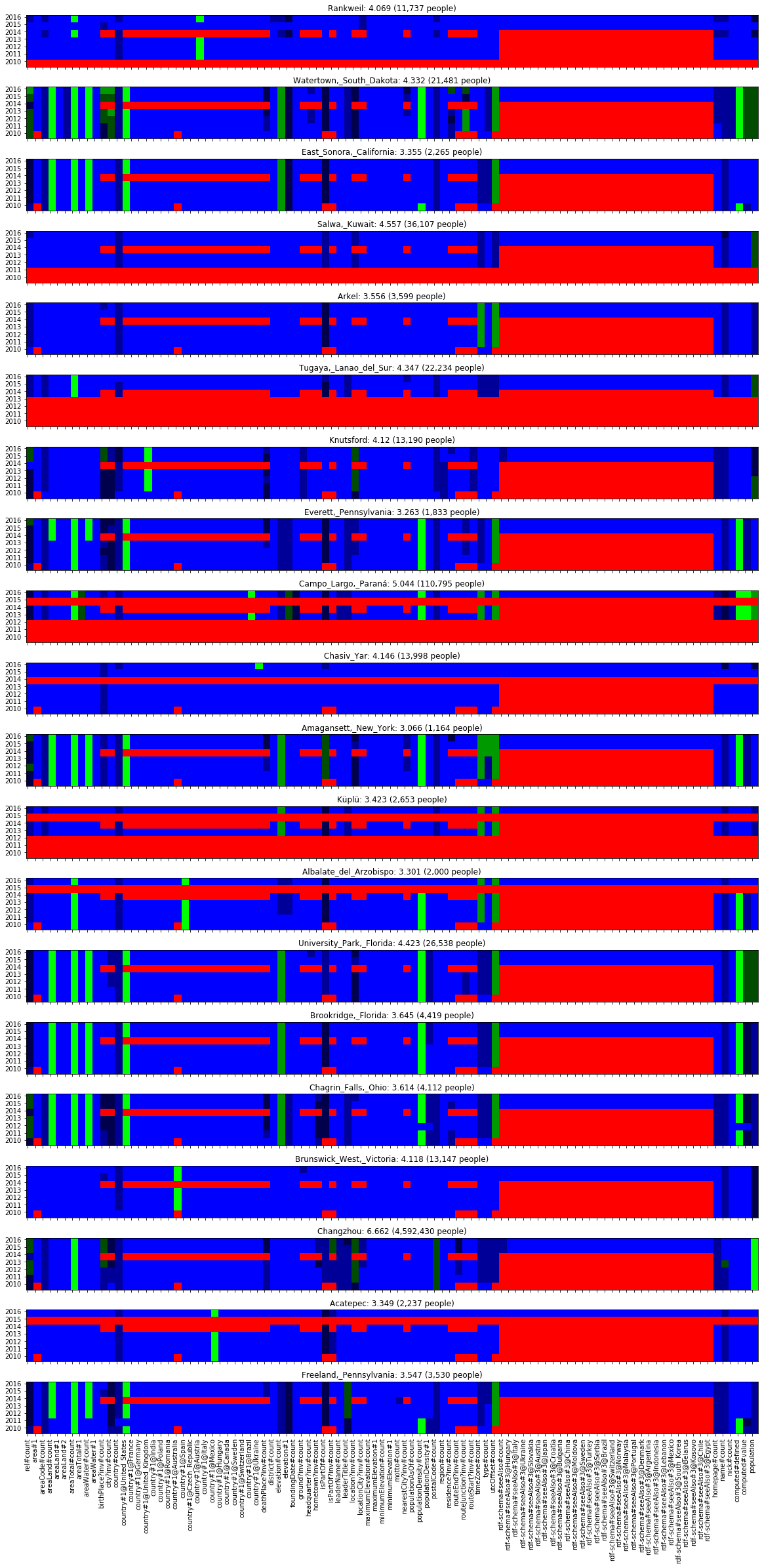From the figure we can see that the relation counts change from version to version but the target population seem to stay within the same range. There are plenty of missing features and the colours indicate a relative growth over time.

The "seeAlso" features are missing for all years, that's a big loss.

## First featurization: time lagged features¶

Given the large percentage of cities that are not present in all years, we will need a way to impute them.

### Imputation¶

I will use the closer available year, given priority to earlier years (Cell #7).

In :
# CELL 7
import random
import math
import numpy as np

rand = random.Random(42)

data = list()
with open("ch7_cell5_dev_missing.tsv") as f:
for line in f:
fields = line.strip().split('\t')
name = fields
feats = list(map(float, fields[1:]))
data.append( (feats, feats[-1], name) )

table = np.zeros( (len(data), len(header)) )
for idx, row in enumerate(data):
table[idx] = row

FEATS = len(base_features)
VERSIONS = 6
CITIES = len(data)

# impute
back_imputed = 0
forward_imputed = 0
present_imputed = 0
for city_idx in range(CITIES):
for version in range(VERSIONS):
for feat in range(FEATS):
if table[city_idx,  2 * FEATS * version + 2 * feat] == 1.0:
continue
lag = 1
imputed = False
while version + lag <= VERSIONS or version - lag >= 0:
if version - lag >= 0 and table[city_idx,  2 * FEATS * (version - lag) + 2 * feat] == 1.0:
table[city_idx,  2 * FEATS * version + 2 * feat + 1] = \
table[city_idx,  2 * FEATS * (version - lag) + 2 * feat + 1]
back_imputed += 1
imputed = True
break
if version + lag < VERSIONS and table[city_idx,  2 * FEATS * (version + lag) + 2 * feat] == 1.0:
table[city_idx,  2 * FEATS * version + 2 * feat + 1] = \
table[city_idx,  2 * FEATS * (version + lag) + 2 * feat + 1]
forward_imputed += 1
imputed = True
break
if version + lag == VERSIONS: # present
table[city_idx,  2 * FEATS * version + 2 * feat + 1] = \
table[city_idx,  2 * FEATS * (version + lag) + feat]
present_imputed += 1
imputed = True
break
lag += 1
if not imputed:
print(version, feat, data[city_idx][-1])

# write full feature table
imputed_cells = 0
total_cells = 0
with open("ch7_cell7_dev_imputed.tsv", "w") as w:
w.write('name')
for city_idx, city in enumerate(map(lambda x:x[-1], data)):
total_cells += FEATS * VERSIONS
w.write(city)
w.write('\t' + '\t'.join(map(str, table[city_idx,:])))
w.write('\n')
imputed_cells = back_imputed + forward_imputed + present_imputed
print("Total cells: {:,} imputed: {:,} ({:%})".format(total_cells, imputed_cells, imputed_cells / total_cells))
print("Backward imputed: {:,} ({:%})".format(back_imputed, back_imputed / imputed_cells))
print("Forward imputed: {:,} ({:%})".format(forward_imputed, forward_imputed / imputed_cells))
print("Present imputed: {:,} ({:%})".format(present_imputed, present_imputed / imputed_cells))

Total cells: 26,705,646 imputed: 11,078,309 (41.483022%)
Backward imputed: 1,770,176 (15.978756%)
Forward imputed: 7,154,111 (64.577644%)
Present imputed: 2,154,022 (19.443599%)


So most features are not present-imputed. That is important because otherwise there will be little value of the historical features.

Let us visualize this (Cell #8).

In :
# CELL 8
import random
import math
import numpy as np
from sklearn.cluster import KMeans

rand = random.Random(42)

data = list()
with open("ch7_cell7_dev_imputed.tsv") as f:
for line in f:
fields = line.strip().split('\t')
name = fields
feats = list(map(float, fields[1:]))
data.append( (feats, feats[-1], name) )

table = np.zeros( (len(data), len(header)) )
for idx, row in enumerate(data):
table[idx] = row

FEATS = len(base_features)
VERSIONS = 6
CITIES = len(data)

# compute boundaries using kMeans
boundaries = list()
for feat in range(FEATS):
col = table[:,2*VERSIONS*FEATS + feat]
vals = set(col)
num_clusters = min(len(vals), 6)
if num_clusters < 6:
boundaries.append(sorted(vals))
else:
kmeans = KMeans(n_clusters=6, random_state=1).fit(col.reshape(-1,1))
boundaries.append(sorted(set(list(kmeans.cluster_centers_.reshape(-1)))))

# save boundaries
with open("ch7_cell8_boundaries.tsv", "w") as b:
for idx, boundary_list in enumerate(boundaries):
b.write("{}\t{}\n".format(idx, "\t".join(map(str,boundary_list))))

# pick some cities at random to graph
to_show = rand.sample(list(range(len(data))), 20)

def cell8_colorize(feat, feat_val, boundaries):
num_classes = len(boundaries[feat])
half_classes = num_classes // 2
if half_classes == 0:
half_classes = 1
dists = sorted(map(lambda x: (abs(x-feat_val), x), enumerate(boundaries[feat])))
clazz = dists
if clazz >= half_classes:
component = 1
clazz -= half_classes
else:
component = 2
clazz = half_classes - clazz - 1

intensity = None
if clazz == 0:
if half_classes == 3:
intensity = 0.3
elif half_classes == 2:
intensity = 0.6
elif half_classes == 1:
intensity = 1.0
elif clazz == 1:
if half_classes == 3:
intensity = 0.6
elif half_classes == 2:
intensity = 1.0
elif half_classes == 1:
intensity = 1.0
elif clazz == 2:
intensity = 1.0
box_color = [ 0.0, 0.0, 0.0 ]
box_color[component] = intensity
#print(dists, clazz, num_classes, half_classes, box_color)
return box_color

HEIGHT=2
WIDTH=2

def cell8_shrink_name(feat_name):
if '@' in feat_name:
parts = feat_name.split('@')
return parts.split("/")[-1] + "@" + parts.split("/")[-1][:-1]
else:
if '/' in feat_name:
return feat_name.split("/")[-1]
return feat_name

img = np.zeros( [ (VERSIONS + 1) * HEIGHT, FEATS * WIDTH, 3 ])
img[:,:,:] = 1.0

yticks_locs = list(map(lambda x:x*HEIGHT, range(VERSIONS+1)))
yticks_labels = list(map(lambda x:2016-x, range(VERSIONS+1)))
xticks_locs = list(map(lambda x:x*WIDTH, range(FEATS)))
xticks_labels = list(map(lambda x:cell8_shrink_name(x), base_features))

from matplotlib import pyplot as plt
%matplotlib inline

plt.rcParams['figure.figsize'] = [20, 40]

def cell8_draw_features(plot, row, title, versions, boundaries,
yticks_locs, yticks_labels, xticks_locs, xticks_labels):
if title is not None:
plot.title(title)
img = np.zeros( [ (versions + 1) * HEIGHT, FEATS * WIDTH, 3 ])
img[:,:,:] = 1.0

xbox = 0
for feat in range(FEATS):
ybox = feat * WIDTH
img[ xbox:(xbox + HEIGHT), ybox: (ybox + WIDTH) ] = \
cell8_colorize(feat, row[2 * versions * FEATS + feat], boundaries)
for version in range(versions):
xbox = (versions - version) * HEIGHT
for feat in range(FEATS):
ybox = feat * WIDTH
box_color = cell8_colorize(feat, row[2 * version * FEATS + 2 * feat + 1], boundaries)
# mark imputation
if row[2 * FEATS * version + 2 * feat] == 0.0:
img[ xbox:(xbox + HEIGHT // 2), ybox: (ybox + WIDTH) ] = [1.0, 0, 0 ]
img[ (xbox + HEIGHT // 2):(xbox + HEIGHT), ybox: (ybox + WIDTH) ] = box_color
else:
img[ xbox:(xbox + HEIGHT), ybox: (ybox + WIDTH) ] = box_color
plot.imshow(img, aspect='equal')
plot.yticks(yticks_locs, yticks_labels)
plot.xticks(xticks_locs, xticks_labels, rotation=90)

plt.axis('on')
for img_idx, idx in enumerate(to_show):
plt.subplot(len(to_show), 1, img_idx + 1)
cell8_draw_features(plt, table[idx,:], "{}: {} ({:,} people)".format(data[idx][-1].split("/")[-1][:-1],
int(table[idx,-1]*1000)/1000.0,
int(10**table[idx,-1])),
VERSIONS, boundaries,
yticks_locs, yticks_labels, xticks_locs,
[] if img_idx < len(to_show) - 1 else xticks_labels)

plt.savefig("ch7_cell8_viz_full.pdf", bbox_inches='tight', dpi=300)